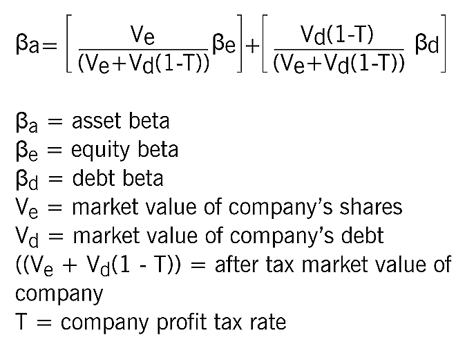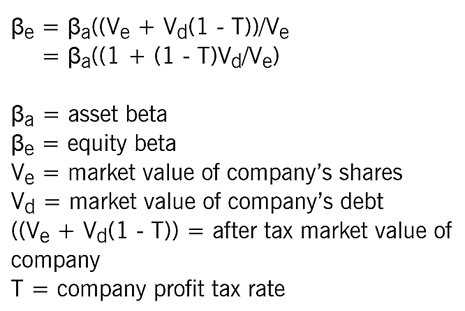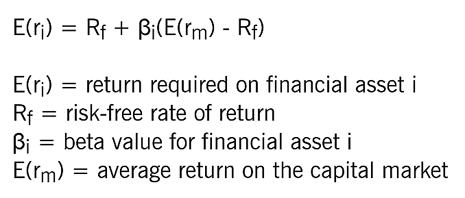The capital asset pricing model – part 2

Project-specific discount rates

Section E of the Study Guide for Financial Management contains several references to the Capital Asset Pricing Model (CAPM). This article, is the second in a series of three, and looks at applying the CAPM in calculating a project-specific discount rate to use in investment appraisal. The first article in the series introduced the CAPM and its components, showed how the model could be used to estimate the cost of equity, and introduced the asset beta formula. The final article will look at the theory, advantages, and disadvantages of the CAPM.

As mentioned in the first article in this series, the CAPM is a method of calculating the return required on an investment, based on an assessment of its risk. When the business risk of an investment project differs from the business risk of the investing company, the return required on the investment project is different from the average return required on the investing company’s existing business operations. This means that it is not appropriate to use the investing company’s existing cost of capital as the discount rate for the investment project. Instead, the CAPM can be used to calculate a project-specific discount rate that reflects the business risk of the investment project.

Proxy companies and proxy betas

The first step in using the CAPM to calculate a project-specific discount rate is to look for companies whose business operations are similar to the proposed investment project. For example, if a food processing company was looking at an investment in coal mining, it would need to locate some coal mining companies. Companies undertaking similar business operations to a proposed investment are known as ‘proxy companies’. Since their equity betas represent the business risk of the proxy companies’ business operations, they are referred to as ‘proxy equity betas’ or ‘proxy betas’.

From a CAPM point of view, these proxy betas can be used to represent the business risk of the proposed investment project. For example, the proxy betas from several coal mining companies ought to represent the business risk of an investment in coal mining.

If you were to look at the equity betas of several coal mining companies, however, it is very unlikely that they would all have the same value. The reason for this is that equity betas reflect not only the business risk of a company’s operations, but also the financial risk of a company. The systematic risk represented by equity betas, therefore, includes both business risk and financial risk.

In the first article in this series, we introduced the idea of the asset beta, which is linked to the equity beta by the asset beta formula. This formula is included in the formulae sheet and is as follows:To proceed with calculating a project-specific discount rate, we need to remove the effect of the financial risk or gearing from each of the proxy equity betas in order to find their asset betas, which are betas that reflect business risk alone. If a company has no gearing, and hence no financial risk, its equity beta and its asset beta have the same value.

Ungearing equity betas

The asset beta formula is a bit unwieldy and so it usual to make the simplifying assumption that the beta of debt  ( β d ) is zero. This is a relatively minor simplification because the debt beta is usually very small compared to the equity beta ( β e ). In addition, the market value of a company’s debt (V d ) is usually very small in comparison to the market value of its equity (V e ), and the tax efficiency of debt reduces the weighting of the debt beta even further.

Assuming the debt beta is zero, the asset beta formula becomes:If the equity beta, the gearing, and the tax rate of the proxy company are known, this amended asset beta formula can be used to calculate the proxy company’s asset beta. Since this calculation removes the effect of the financial risk or gearing of the proxy company from the proxy beta, it is usually called ‘ungearing the equity beta’. Similarly, the amended asset beta formula is called the ‘ungearing formula’.

Averaging asset betas

After the equity betas of several proxy companies have been ungeared, it is usually found that the resulting asset betas have slightly different values. This is not that surprising, since it is very unlikely that two proxy companies will have identical systematic business risk. Even two coal mining companies will not be mining the same coal seam, or mining the same kind of coal, or selling coal into the same market. If one of the calculated asset betas is very different from the others, however, it would be regarded with suspicion and excluded from further consideration.

To remove the effect of the slight differences in business operations and business risk that are reflected in the asset betas, these asset betas are averaged. A simple arithmetic mean is calculated by adding up the asset betas and then dividing by the number of asset betas being averaged.

Regearing the asset beta

The average asset beta represents the business risk of the proposed investment project. Before a project-specific discount rate can be calculated, however, the financial risk of the investing company needs to be taken into consideration. In other words, having ungeared the proxy equity betas when calculating the asset betas, it is now necessary to ‘regear’ the average asset beta to reflect the gearing and the financial risk of the investing company.

One way to approach regearing is to use the ungearing formula, inserting the gearing and the tax rate of the investing company, and the average asset beta, and leaving the equity beta as the only unknown variable. Another approach is to rearrange the ungearing formula in order to represent the equity beta in terms of the asset beta, as follows:The gearing and the tax rate of the investing company, and the average asset beta, are inserted into the right-hand side of the regearing formula to calculate the regeared equity beta.

Calculating the project-specific discount rate

The CAPM can now be used to calculate a project-specific cost of equity. Once values have been obtained for the risk-free rate of return, and either the equity risk premium or the return on the market, these can be inserted into the CAPM formula along with the regeared equity beta:The project-specific cost of equity can be used as the project-specific discount rate or project-specific cost of capital. It is also possible to go further and calculate a project-specific weighted average cost of capital, but this does not concern us in this article and it is a step that is often omitted when using the CAPM in investment appraisal.

Summary of steps in the calculation

The steps in calculating a project-specific discount rate using the CAPM can now be summarised, as follows:

1. Locate suitable proxy companies.
2. Determine the equity betas of the proxy companies, their gearings and tax rates.
3. Ungear the proxy equity betas to obtain asset betas.
4. Calculate an average asset beta.
5. Regear the asset beta.
6. Use the CAPM to calculate a project-specific cost of equity.

The difficulties and practical problems associated with using the CAPM to calculate a project-specific discount rate to use in investment appraisal will be discussed in the next article in this series.

EXAMPLE 1

Lad Co is planning to invest in a new project that is significantly different from its existing business operations. The company is financed 30% by debt and 70% by equity. It has identified three companies whose business operations are similar to the proposed investment, and details of these companies are as follows:

• Cup Co has an equity beta of 0.81 and  financed 25% by debt and 75% by equity.
• Mug Co has an equity beta of 0.98 and  financed 40% by debt and 60% by equity.
• Jug Co has an equity beta of 1.16 and  financed 50% by debt and 50% by equity.

Assume that the risk-free rate of return is 4% per year, and that the equity risk premium is 6% per year. Assume also that all the companies pay tax at a rate of 25% per year. Calculate a project-specific discount rate for the proposed investment.

Solution

Ungearing the proxy equity betas:

• Cup Co asset beta  = 0.81 x 75/(75 + 25(1 – 0.25)) = 0.648
• Mug Co asset beta = 0.98 x 60/(60 + 40(1 – 0.25)) = 0.653
• Jug Co asset beta = 1.16 x 50/(50 + 50(1 – 0.25)) = 0.663

Averaging the asset betas:
(0.648 + 0.653 + 0.663)/3 = 1.964/3 = 0.655

Regearing the average asset beta: 0.655 = βe x 70/(70 + 30(1 – 0.25)) = βe x 0.757.

Hence βe = 0.655/0.757 = 0.865

If the regearing equation were used:

βe = 0.655 x (1 + (1 – 0.25)30/70) = 0.866

Calculating the project-specific discount rate:

E(ri) = Rf + βi (E(rm) – Rf) = 4 + (0.865 x 6) = 4 + 5.19 = 9.2%

Written by a member of the Financial Management examining team